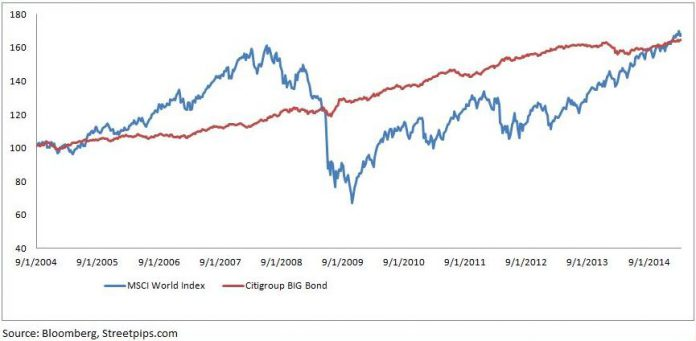# Live To Trade Another Day

534In traditional investing, risk is viewed as volatility or standard deviation of the asset’s marked to market value. Standard deviation tells you how much an investment’s value will fluctuate from the average return. The more volatile the investment is likely to swing (both positively and negatively) around it’s own historical average, the more risky an investment or asset class is.

For example, Chart 1 below shows the normalised returns of Global Equities, as represented by MSCI World Index, versus Global Bonds, as represented by Citigroup Broad Investment Grade Bonds Index.

If you had invested \$100 in both Global Equities and Global Bonds for the past 10 years, your portfolio value of either would be fairly similar, which is \$168 for Global Equities and \$165 for Global Bonds. (pretty dismal annualised returns per unit risk for Global Equities but that’s another story altogether) However, Global Equities is more volatile than Global Bonds and hence more risky by definition. The standard deviation of a time series of daily returns for Global Equities is 2.53% versus 0.50% for Global Bonds.

Chart 1: Normalised Returns For Past 10 Years For Global Equities & Global Bonds

There are many more measures of traditional measures of risk such as Value-at-Risk or Conditional-Value-at-Risk which is an extension of VAR. These measures permeate the vast majority of investors for many years now.

However the most important and relevant risk to me when I trade is the Risk of Ruin. There were many sources of inspiration and influences (the “Market Wizards” type of traders and successful fund managers) through the years in shaping my thoughts on trading and this concept of looking at risk as the “risk of losses of trading capital” has been one of the most important.

So how do we quantify the risk of ruin? I came across these 2 methods as described below. They were referenced from D.R. Cox and H.D Miller in “The Theory of Stochastic Processes”.

For fixed trade size (e.g. 1 lot per trade)

R= Risk of losing z fraction of the trading capital

e = Base of natural logarithm, 2.71828

z = If we want to calculate the risk of losing half the account, input 0.5

a = mean return of the trades, must be same time frame as d. For example if daily mean returns are used, then use standard deviation of daily returns. If weekly mean returns are used, then use standard deviation of weekly returns.

d = standard deviation of returns, must be same time frame as mean returns mentioned earlier.

For fixed trade percentage (e.g. 2% of capital per trade)R= Risk of losing z fraction of the trading capital

e = Base of natural logarithm, 2.71828

ln(1-z) = natural logarithm of (1-z)

z = If we want to calculate the risk of losing half the account, input 0.5

a = mean return of the trades, must be same time frame as d. For example if daily mean returns are used, then use standard deviation of daily returns. If weekly mean returns are used, then use standard deviation of weekly returns.

d = standard deviation of returns, must be same time frame as mean returns mentioned earlier.

You may wish to incorporate these calculations in your money management tools to give you an idea of the risk of ruin which is so important in trading. Live to trade another day. It is all about survival in this game!